# Lathyrus vernus function-based age-by-stage MPMs

In this vignette, we use the lathyrus dataset to illustrate the estimation of age-by-stage function-based MPMs. These are inherently ahistorical MPMs, and so we do not include historical analyses in this vignette. To reduce output size, we have prevented some statements from running if they produce long stretches of output. Examples include most summary() calls. In these cases, we include hashtagged versions of these calls, and our text assumes that the user runs these statements without hashtags. This vignette is only a sample analysis. Detailed information and instructions on using lefko3 are available through a free online e-book called lefko3: a gentle introduction, available on the projects page of the Shefferson lab website. This website also includes links to long-format vignettes and other demonstrations of this package.

### ORGANISM AND POPULATION

Lathyrus vernus (family Fabaceae) is a long-lived forest herb, native to Europe and large parts of northern Asia. Individuals increase slowly in size and usually flower only after 10-15 years of vegetative growth. Flowering individuals have an average conditional lifespan of 44.3 years (Ehrlén and Lehtila 2002). L. vernus lacks organs for vegetative spread and individuals are well delimited (Ehrlén 2002). One or several erect shoots of up to 40 cm height emerge from a subterranean rhizome in March-April. Flowering occurs about four weeks after shoot emergence. Shoot growth is determinate, and the number of flowers is determined in the previous year (Ehrlén and Van Groenendael 2001). Individuals may not produce aboveground structures every year, but can remain dormant in one or more seasons. L. vernus is self-compatible but requires visits from bumble-bees to produce seeds. Individuals produce few, large seeds and establishment from seeds is relatively frequent (Ehrlén and Eriksson 1996). The pre-dispersal seed predator Bruchus atomarius often consumes a large fraction of developing seeds, and roe deer (Capreolus capreolus) sometimes consume the shoots (Ehrlén and Munzbergova 2009).

Data for this study were collected from six permanent plots in a population of L. vernus located in a deciduous forest in the Tullgarn area, SE Sweden (58.9496 N, 17.6097 E), during 1988–1991 (Ehrlén 1995). The six plots were similar with regard to soil type, elevation, slope, and canopy cover. Within each plot, all individuals were marked with numbered tags that remained over the study period, and their locations were carefully mapped. New individuals were included in the study in each year. Individuals were recorded at least three times every growth season. At the time of shoot emergence, we recorded whether individuals were alive and produced above-ground shoots, and if shoots had been grazed. During flowering, we recorded flower number and the height and diameter of all shoots. At fruit maturation, we counted the number of intact and damaged seeds. To derive a measure of above-ground size for each individual, we calculated the volume of each shoot as $$\pi × (\frac{1}{2} diameter)^2 × height$$, and summed the volumes of all shoots. This measure is closely correlated with the dry mass of aboveground tissues ($$R^2 = 0.924$$, $$P < 0.001$$, $$n = 50$$, log-transformed values; Ehrlén 1995). Size of individuals that had been grazed was estimated based on measures of shoot diameter in grazed shoots, and the relationship between shoot diameter and shoot height in non-grazed individuals. Only individuals with an aboveground volume of more than 230 mm3 flowered and produced fruits during this study. Individuals that lacked aboveground structures in one season but reappeared in the following year were considered dormant. Individuals that lacked aboveground structures in two subsequent seasons were considered dead from the year in which they first lacked aboveground structures. Probabilities of seeds surviving to the next year, and of being present as seedlings or seeds in the soil seed bank, were derived from separate yearly sowing experiments in separate plots adjacent to each subplot (Ehrlén and Eriksson 1996).

### BASIC WORKFLOW

Our goal in this exercise will be to produce ahistorical, age-by-stage function-based matrices using the Lathyrus dataset. We will use a different approach to size classification than in previous vignettes, using the natural log of size instead of the normal size shown in the dataset. Our reasoning has to do with the fact that volume is used as the size metric here, and so should have an allometric relationship to some vital rates (note that all size metrics have allometric relationships, but this is clearer when size is based on something more strongly related to mass, as is volume). We will also create both reproductive and non-reproductive stages of the same size classes.

The dataset that we have provided is organized in horizontal format, meaning that each row holds all of the data for a single, unique individual, and columns correspond to individual condition in particular monitoring occasions (which we refer to as years here, since there was one main census in each year). The original spreadsheet file used to keep the dataset has a repeating pattern to these columns, with each year having a similarly arranged group of variables. Package lefko3 includes functions to handle data in horizontal format, as well as vertically formatted data (i.e. data for individuals is broken up across rows, where each row is a unique combination of individual and year in occasion t). Let’s load the dataset.

data(lathyrus)
#summary(lathyrus)

This dataset includes information on 1,119 individuals, so there are 1,119 rows with data (not counting the header). There are 38 columns. The first two columns are variables identifying each individual (SUBPLOT refers to the patch, and GENET refers to individual identity), with each individual’s data entirely restricted to one row. This is followed by four sets of nine columns, each named VolumeXX, lnVolXX, FCODEXX, FlowXX, IntactseedXX, Dead19XX, DormantXX, Missing19XX, and SeedlingXX, where XX corresponds to the year of observation and with years organized consecutively. Thus, columns 3-11 refer to year 1988, columns 12-20 refer to year 1989, etc. This strictly repeated pattern allows us to manipulate the original dataset quickly and efficiently via lefko3. We should know the total number of years of data in the dataset, which is four years here (includes all years from and including 1988 to 1991). Ideally, we should also have arranged the columns in the same order for each year, with years in consecutive order with no extra columns between them. Note that this order is not required, but it makes life easier because following a strictly repeating pattern allows us to skip inputting the names or numbers of each column of data directly later during demographic data formatting (step 2a below).

#### Step 1. Life history model development

To begin, we will create a stageframe for this dataset. A stageframe is a data frame that describes all stages in the life history of the organism, in a way usable by the functions in this package and using stage names and classifications that completely match those used in the dataset. It links the dataset and our analyses to our life history model. In this case, the life history model is a life cycle graph (Figure 8.1). This model is based on the life history model provided in Ehrlén (2000), but we utilize a different size classification based on the log leaf volume to make the size distribution more closely match a symmetric and somewhat normal distribution.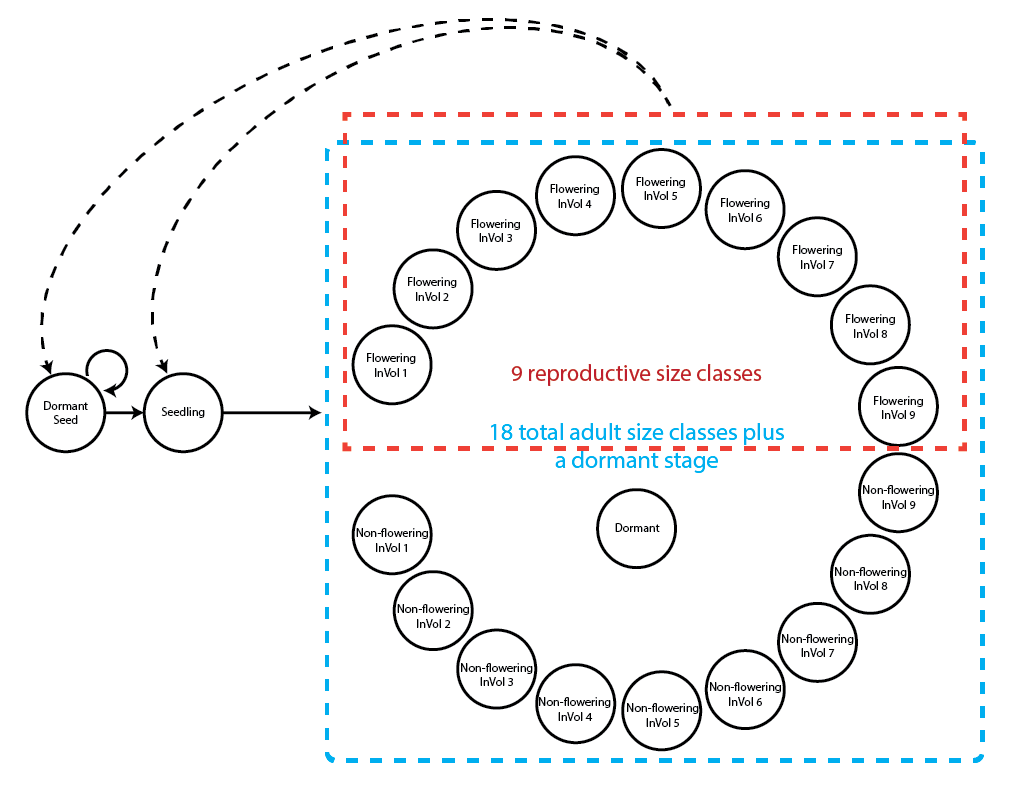Figure 8.1. Life history model of Lathyrus vernus using the log leaf volume as the size classification metric.

Our stageframe needs to include complete descriptions of all stages that occur in the dataset, with each stage defined uniquely, and also needs to describe for each stage portrayed in our life history model. Since this object can be used for automated classification of individuals, all sizes, reproductive states, and other characteristics defining each stage in the dataset need to be accounted for explicitly. However, combinations of life history characteristics that appear to be outliers should be excluded, as they can lead to errors and issues in inference. So, great care must be taken to include all size values and values of other descriptor variables occurring within the dataset. The final description of each stage occurring in the dataset must not completely overlap with any other stage also found in the dataset, although partial overlap is allowed and expected.

Before creating the stageframe, let’s explore the possible size variables. We will particularly look at summaries of the distribution of original and log sizes.

summary(c(lathyrus$Volume88, lathyrus$Volume89, lathyrus$Volume90, lathyrus$Volume91))
#>    Min. 1st Qu.  Median    Mean 3rd Qu.    Max.    NA's
#>     1.8    14.7   123.0   484.2   732.5  7032.0    1248
summary(c(lathyrus$lnVol88, lathyrus$lnVol89, lathyrus$lnVol90, lathyrus$lnVol91))
#>    Min. 1st Qu.  Median    Mean 3rd Qu.    Max.    NA's
#>   0.600   2.700   4.800   4.777   6.600   8.900    1248

The upper summary shows the original size, while the lower line shows the size given in logarithmic terms. We should note the size minima and maxima, because we have been using 0 as the size of vegetatively dormant individuals. The lowest uncorrected size is 1.8, with a maximum of 7032. The minimum corrected size is 0.6, and the maximum corrected size is 8.9. Since the minimum corrected size is positive, and since the number of NAs has not increased (increased NAs would suggest that some unusable log sizes occur in the dataset), we are still able to use the log size value 0 as an indicator of vegetative dormancy. Note, however, that vegetative dormancy is currently included in the many NAs that occur in size variables in this dataset.

It can also help to take a look at plots of these distributions. We will plot raw and log volume.

par(mfrow=c(1,2))
plot(density(c(lathyrus$Volume88, lathyrus$Volume89, lathyrus$Volume90, lathyrus$Volume91), na.rm = TRUE), main = "", xlab = "Volume", bty = "n")
plot(density(c(lathyrus$lnVol88, lathyrus$lnVol89, lathyrus$lnVol90, lathyrus$lnVol91), na.rm = TRUE), main = "", xlab = "Log volume", bty = "n")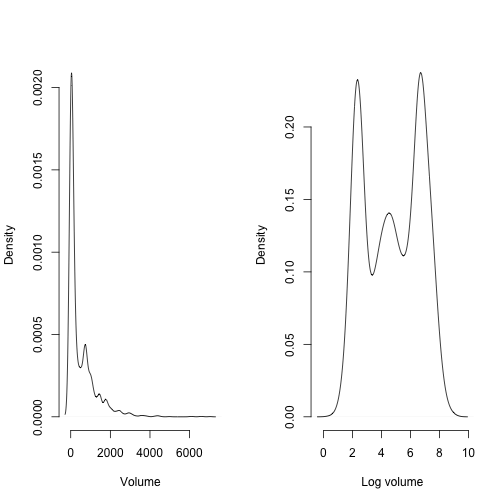Figure 8.2. Density plot of aboveground plant volume

Note how highly skewed the raw volume distribution is. This might cause some difficulty if we used raw size untransformed and with a Gaussian distribution. Certainly, a Gamma distribution would be perfectly justified, and users are urged to try that approach. We will use the log volume here, which looks ‘better’ than the raw volume distribution in the sense that it is closer to some semblance of a Gaussian distribution, mostly through an increased level of symmetry. We can then assume that log volume is Gaussian-distributed and that the mean bears no relationship to the variance.

We will now develop a stageframe that incorporates the log volume of size. We will build this by creating vectors of the values describing each stage, always in the same order.

sizevector <- c(0, 4.6, 0, 1, 2, 3, 4, 5, 6, 7, 8, 9, 1, 2, 3, 4, 5, 6, 7, 8, 9)
stagevector <- c("Sd", "Sdl", "Dorm", "Sz1nr", "Sz2nr", "Sz3nr", "Sz4nr",
"Sz5nr","Sz6nr", "Sz7nr", "Sz8nr", "Sz9nr", "Sz1r", "Sz2r", "Sz3r", "Sz4r",
"Sz5r", "Sz6r", "Sz7r", "Sz8r", "Sz9r")
repvector <- c(0, 0, 0, 0, 0, 0, 0, 0, 0, 0, 0, 0, 1, 1, 1, 1, 1, 1, 1, 1, 1)
obsvector <- c(0, 1, 0, 1, 1, 1, 1, 1, 1, 1, 1, 1, 1, 1, 1, 1, 1, 1, 1, 1, 1)
matvector <- c(0, 0, 1, 1, 1, 1, 1, 1, 1, 1, 1, 1, 1, 1, 1, 1, 1, 1, 1, 1, 1)
immvector <- c(1, 1, 0, 0, 0, 0, 0, 0, 0, 0, 0, 0, 0, 0, 0, 0, 0, 0, 0, 0, 0)
propvector <- c(1, 0, 0, 0, 0, 0, 0, 0, 0, 0, 0, 0, 0, 0, 0, 0, 0, 0, 0, 0, 0)
indataset <- c(0, 1, 1, 1, 1, 1, 1, 1, 1, 1, 1, 1, 1, 1, 1, 1, 1, 1, 1, 1, 1)
binvec <- c(0, 4.6, 0.5, 0.5, 0.5, 0.5, 0.5, 0.5, 0.5, 0.5, 0.5, 0.5, 0.5, 0.5,
0.5, 0.5, 0.5, 0.5, 0.5, 0.5, 0.5)
minima <- c(0, 0, 1, 1, 1, 1, 1, 1, 1, 1, 1, 1, 1, 1, 1, 1, 1, 1, 1, 1, 1)
maxima <- c(NA, 1, NA, NA, NA, NA, NA, NA, NA, NA, NA, NA, NA, NA, NA, NA,
NA, NA, NA, NA, NA)
comments <- c("Dormant seed", "Seedling", "Dormant", "Size 1 Veg", "Size 2 Veg",
"Size 3 Veg", "Size 4 Veg", "Size 5 Veg", "Size 6 Veg", "Size 7 Veg",
"Size 8 Veg", "Size 9 Veg", "Size 1 Flo", "Size 2 Flo", "Size 3 Flo",
"Size 4 Flo", "Size 5 Flo", "Size 6 Flo", "Size 7 Flo", "Size 8 Flo",
"Size 9 Flo")
lathframeln <- sf_create(sizes = sizevector, stagenames = stagevector,
repstatus = repvector, obsstatus = obsvector, propstatus = propvector,
immstatus = immvector, matstatus = matvector, indataset = indataset,
binhalfwidth = binvec, minage = minima, maxage = maxima, comments = comments)
#lathframeln

#### Step 2a. Data standardization

Once the stageframe is created, we can standardize the dataset into historically-formatted vertical (hfv) format. Here, ‘vertical’ format is a way of organizing demographic data in which each row corresponds to the state of a single individual in two (if ahistorical) or three (if historical) consecutive monitoring occasions. To handle this, we will use the verticalize3() function, which creates historically-formatted vertical datasets, as below. We will also replace NAs in size and fecundity variables with zeros for modelsearch to work properly when we build models of vital rates, so we will now set NAas0 = TRUE. Some care needs to be taken with this last step, since some authors give missing values extra meaning not present in a value of zero. In our case, a missing value indicates that a plant was dead (both size and fecundity are missing), was alive but not sprouting (size was missing), or was alive but did not produce seed (fecundity was missing). In all cases, these NA values may be replaced by 0, because other variables indicate those conditions.

We also have two choices for use as our reproductive status and fecundity variables. The first choice, FCODE88 indicates whether a plant flowered. The second choice, Intactseed88, indicates the number of seed produced. Flowering plants typically have different demographic characteristics than non-flowering plants, either reflecting reproductive costs, or, conversely, because flowering plants might have more resources and hence higher survival than non-flowering plants. We should separate transitions among these two groups. So, let’s use FCODE88 to indicate reproductive status, and Intactseed88 to indicate fecundity.

Finally, note that in the input to the following function, we utilize a strictly repeating pattern of variable names arranged in the same order for each monitoring occasion. This arrangement allows us to enter only the first variable in each set, as long as noyears and blocksize are set properly and no gaps or shuffles appear in the dataset. The data management functions that we have created for lefko3 do not require such repeating patterns, but they do make the required input in the function much shorter and more succinct. To see a detailed summary of the resulting hfv dataset, remove full = FALSE from the summary_hfv() call.

lathvertln <- verticalize3(lathyrus, noyears = 4, firstyear = 1988,
patchidcol = "SUBPLOT", individcol = "GENET", blocksize = 9,
juvcol = "Seedling1988", sizeacol = "lnVol88", repstracol = "FCODE88",
nonobsacol = "Dormant1988", stageassign = lathframeln,
stagesize = "sizea", censorcol = "Missing1988", censorkeep = NA,
NAas0 = TRUE, censor = TRUE)
#> hfv dataset has been censored using a non-repeating censor variable.
#>       Please check the resulting dataset with the summary_hfv() function to make
#>       sure that not too much has been removed from the original dataset.
summary_hfv(lathvertln, full = FALSE)
#>
#> This hfv dataset contains 2552 rows, 42 variables, 1 population, 6 patches, 276 individuals, and 3 time steps.

Before we move on to the next key steps in analysis, let’s take a closer look at fecundity. In this dataset, fecundity is mostly a count of intact seeds, and only differs in six cases where the seed output was estimated based on other models. To see this, try the following code.

writeLines(paste0("Length of fecundity vaiable in t+1: ", length(lathvertln$feca3))) #> Length of fecundity vaiable in t+1: 2552 writeLines(paste0("# non-integer entries: ", length(which(lathvertln$feca3 !=
round(lathvertln$feca3))))) #> # non-integer entries: 0 writeLines(paste0("Length of fecundity variable in t: ", length(lathvertln$feca2)))
#> Length of fecundity variable in t: 2552
writeLines(paste0("# non-integer entries: ", length(which(lathvertln$feca2 != round(lathvertln$feca2)))))
#> # non-integer entries: 6
writeLines(paste0("Length of fecundity variable in t-1: ", length(lathvertln$feca1))) #> Length of fecundity variable in t-1: 2552 writeLines(paste0("# non-integer entries: ", length(which(lathvertln$feca1 !=
round(lathvertln$feca1))))) #> # non-integer entries: 6 We see that we have quite a bit of fecundity data, and that it is overwhelmingly but not exclusively composed of integer values. The six non-integer values force us to make a decision - should we treat fecundity as a continuous variable, or round the values and treat it as a count variable? This is actually a vital decision that will strongly affect our vital rate model of fecundity, because treating it as continuous will also imply particular relationships between the mean and the variance. Here, we will round fecundity so that we can treat fecundity as a count variable in the analysis. lathvertln$feca3 <- round(lathvertln$feca3) lathvertln$feca2 <- round(lathvertln$feca2) lathvertln$feca1 <- round(lathvertln$feca1) The fact that fecundity is now integer-based suggests that it can be treated as a count variable. This package currently allows eight choices of count distributions: Gaussian, Gamma, Poisson, negative binomial, zero-inflated Poisson, zero-inflated negative binomial, zero-truncated Poisson, and zero-truncated negative binomial. To assess which to use, we should first assess whether the mean and variance of the count are equal using a dispersion test. This test allows us to test whether the variance is greater than that expected under our mean value for fecundity using a chi-squared test. If it is not significantly different, then we may use some variant of the Poisson distribution. If the data are overdispersed, then we should use the negative binomial distribution. We should also test whether the number of zeroes is more than expected under these distributions, and make the distribution zero-inflated if so. Note that, because we have not excluded zeros from fecundity using reproductive status, we should not use a zero-truncated distribution. Let’s start off by looking at a bar plot of the distribution of fecundity. hist(subset(lathvertln, repstatus2 == 1)$feca2, main = "Fecundity",
xlab = "Intact seeds produced in occasion t")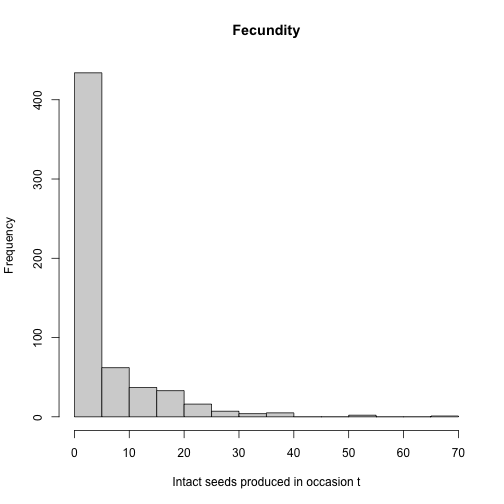Figure 8.3. Histogram of fecundity in occasion t

We see that the distribution conforms to a classic count variable with a very low mean value. The first bar suggests that there may be too many zeroes, as well. Let’s now formally test our assumptions. We will use the hfv_qc() function, which will allow us to assess the quality of the data from the viewpoint of the linear models to be built later. We will use most of the input from the modelsearch() call that we will conduct later.

hfv_qc(data = lathvertln, historical = FALSE, suite = "main",
vitalrates = c("surv", "obs", "size", "repst", "fec"), juvestimate = "Sdl",
indiv = "individ", year = "year2", age = "obsage", year.as.random = TRUE,
patch.as.random = TRUE)
#> Survival:
#>
#>   Data subset has 43 variables and 2267 transitions.
#>
#>   Variable alive3 has 0 missing values.
#>   Variable alive3 is a binomial variable.
#>
#>
#> Observation status:
#>
#>   Data subset has 43 variables and 2122 transitions.
#>
#>   Variable obsstatus3 has 0 missing values.
#>   Variable obsstatus3 is a binomial variable.
#>
#>
#> Primary size:
#>
#>   Data subset has 43 variables and 1916 transitions.
#>
#>   Variable sizea3 has 0 missing values.
#>   Variable sizea3 appears to be a floating point variable.
#>   1753 elements are not integers.
#>   The minimum value of sizea3 is 1.2 and the maximum is 8.8.
#>   The mean value of sizea3 is 5.099 and the variance is 3.093.
#>   The value of the Shapiro-Wilk test of normality is 0.9551 with P = 7.719e-24.
#>   Variable sizea3 differs significantly from a Gaussian distribution.
#>
#>   Variable sizea3 is fully positive, lacking even 0s.
#>
#>
#> Reproductive status:
#>
#>   Data subset has 43 variables and 1916 transitions.
#>
#>   Variable repstatus3 has 0 missing values.
#>   Variable repstatus3 is a binomial variable.
#>
#>
#> Fecundity:
#>
#>   Data subset has 43 variables and 601 transitions.
#>
#>   Variable feca2 has 0 missing values.
#>   Variable feca2 appears to be an integer variable.
#>
#>   Variable feca2 is fully non-negative.
#>
#>   Overdispersion test:
#>     Mean feca2 is 4.805
#>     The variance in feca2 is 70.23
#>     The probability of this dispersion level by chance assuming that
#>     the true mean feca2 = variance in feca2,
#>     and an alternative hypothesis of overdispersion, is 0
#>     Variable feca2 is significantly overdispersed.
#>
#>   Zero-inflation and truncation tests:
#>     Mean lambda in feca2 is 0.008186
#>     The actual number of 0s in feca2 is 335
#>     The expected number of 0s in feca2 under the null hypothesis is 4.92
#>     The probability of this deviation in 0s from expectation by chance is 0
#>     Variable feca2 is significantly zero-inflated.
#>
#>
#> Juvenile survival:
#>
#>   Data subset has 43 variables and 285 transitions.
#>
#>   Variable alive3 has 0 missing values.
#>   Variable alive3 is a binomial variable.
#>
#>
#> Juvenile observation status:
#>
#>   Data subset has 43 variables and 210 transitions.
#>
#>   Variable obsstatus3 has 0 missing values.
#>   Variable obsstatus3 is a binomial variable.
#>
#>
#> Juvenile primary size:
#>
#>   Data subset has 43 variables and 193 transitions.
#>
#>   Variable sizea3 has 0 missing values.
#>   Variable sizea3 appears to be a floating point variable.
#>   183 elements are not integers.
#>   The minimum value of sizea3 is 0.7 and the maximum is 4.1.
#>   The mean value of sizea3 is 2.307 and the variance is 0.2051.
#>   The value of the Shapiro-Wilk test of normality is 0.9273 with P = 3.278e-08.
#>   Variable sizea3 differs significantly from a Gaussian distribution.
#>
#>   Variable sizea3 is fully positive, lacking even 0s.
#>
#>
#> Juvenile reproductive status:
#>
#>   Data subset has 43 variables and 193 transitions.
#>
#>   Variable repstatus3 has 0 missing values.
#>   Variable repstatus3 is a binomial variable.
#>
#>
#> Juvenile maturity status:
#>
#>   Data subset has 43 variables and 210 transitions.
#>
#>   Variable matstatus3 has 0 missing values.
#>   Variable matstatus3 is a binomial variable.

All of the probability variables look like binomials, so we have no problem there. Size and juvenile size appear to be continuous variables that differ significantly from the assumptions of the Gaussian distribution. However, we will still use a Gaussian distribution in this case, for instructional purposes. Fecundity is a count variable with significant overdispersion and significantly more zeros than expected, so we should use a zero-inflated negative binomial distribution.

#### Step 2b. Provide supplemental information for matrix estimation

Matrix estimation functions in package lefko3 are made to work with supplement tables, which provide extra data for matrix estimation that is not included in the main demographic dataset. The supplemental() function provides a means of incorporating four additional kinds of data into MPM construction:

1. fixed transition values derived from other studies and added as constants to matrices,
2. proxy transition values when data for particular transitions do not exist and other, estimable transitions need to be used as proxies,
3. reproductive multipliers indicating which stages lead to the production of which stages, and at what level relative to estimated fecundity, as well as multipliers for proxy transitions, and
4. survival multipliers, in cases in which proxy survival transitions are used but must be set at elevated or reduced levels relative to the original transition.

Here, we will create an ahistorical supplemental table organizing some of these sorts of data. Each row refers to a specific transition. The first two of these transitions are set to specific probabilities, which are the probabilities of germination and seed dormancy, estimated from a separate study. The final two terms are fecundity multipliers, which mark which transitions correspond to fecundity and provide information on what multiple of fecundity estimated via linear modeling applies to each case.

lathsupp2 <- supplemental(stage3 = c("Sd", "Sdl", "Sd", "Sdl"),
stage2 = c("Sd", "Sd", "rep", "rep"),
givenrate = c(0.345, 0.054, NA, NA),
multiplier = c(NA, NA, 0.345, 0.054),
type = c(1, 1, 3, 3), stageframe = lathframeln, historical = FALSE)
#lathsupp2

These supplement tables provide the best means of adding external data to our MPMs because they allow both specific transitions to be isolated, and because they allow the use of shorthand to identify large groups of transitions (e.g. using mat, immat, rep, nrep, prop, npr, obs, nobs, groupX, or all to signify all mature stages, immature stages, reproductive stages, non-reproductive stages, propagule stages, non-propagule stages, observable stages, unobservable stages, stages within group X, or simply all stages, respectively). They also allow more powerful changes to MPMs, such as block reset of groups of transitions to zero or other constants.

#### Step 3. Tests of history, and vital rate modeling

Next we will run the modelsearch function with the new vertical dataset. This function will develop our best-fit vital rate models for us. This function looks simple, but it automates several crucial and complex tasks in MPM analysis. Specifically, it automates 1) the building of global models for each vital rate requested, 2) the exhaustive construction of all reduced models, and 3) the selection of the best-fit models.

Let’s look at just some of the options that we can utilize for this purpose.

historical: Setting this to TRUE or FALSE indicates to include state in occasion t-1 in the global models.

approach: Designates whether to use generalized linear models (glm), in which all factors are treated as fixed, or mixed effects models (mixed), in which factors are treated as either fixed or random (most notably, time, patch, and individual identity can be treated as random).

suite: Designates which factors to include in the global model. Possible values include size, which includes size only; rep, which includes reproductive status only; main, which includes both size and reproductive status as main effects only; full, which includes both size and reproductive status and all two-way interactions; and cons, which includes only an intercept. These factors are in addition to individual identity, patch, and time.

vitalrates: Designates which vital rates to model. The default is to model survival, size, and fecundity, but users can also model observation status and reproduction status or drop some terms.

juvestimate: Designates the name of the juvenile stage transitioning to maturity, in cases where the dataset includes data on juveniles.

juvsize: Denotes whether size should be used as an independent term in models involving transition from the juvenile stage.

bestfit: Denotes whether the best-fit model should be chosen as the model with the lowest AICc (AICc) or as the most parsimonious model (AICc&k), where the latter is the model that has the fewest estimated parameters and is within 2 AICc units of the model with the lowest AICc.

sizedist: Designates the distribution used for size. The options include gaussian, gamma, poisson, and negbin, the last of which refers to the negative binomial distribution (quadratic parameterization). Versions also exist for up to two further size variables in addition to primary size.

ipm_method: Designates whether to estimate size transition probabilities using the cumulative density function (CDF) method (the default), or the midpoint method. The CDF method is exact while the midpoint method generally overestimates (see Doak et al. (2021) for further details).

fecdist: Designates the distribution used for fecundity. The options include gaussian, gamma, poisson, and negbin, the last of which refers to the negative binomial distribution (quadratic parameterization).

fectime: Designates whether fecundity is estimated within occasion t (the default) or occasion t+1. Plant ecologists are likely to choose the former, since fecundity is typically estimated via a proxy such as flowers, fruit, or seed produced. Wildlife ecologists might choose the latter, since fecundity may be best estimated as a count of actual juveniles within nests, burrows, or other family structures.

size.zero: Designates whether the size distribution should be zero-inflated. Only applies to the Poisson and negative binomial distributions. Versions also exist for up to two further size variables in addition to primary size.

size.trunc: Designates whether to use a zero-truncated distribution for size. Only applies to the Poisson and negative binomial distributions, and cannot be applied if size.zero = TRUE. Versions also exist for up to two further size variables in addition to primary size.

fec.zero: Designates whether the fecundity distribution should be zero-inflated. Only applies to the Poisson and negative binomial distributions.

fec.trunc: Designates whether to use a zero-truncated distribution for fecundity. Only applies to the Poisson and negative binomial distributions, and cannot be applied if fec.zero = TRUE.

jsize.zero: Designates whether the juvenile size distribution should be zero-inflated. Only applies to the Poisson and negative binomial distributions. Versions also exist for up to two further size variables in addition to primary size.

jsize.trunc: Designates whether to use a zero-truncated distribution for juvenile size. Only applies to the Poisson and negative binomial distributions, and cannot be applied if jsize.zero = TRUE. Versions also exist for up to two further size variables in addition to primary size.

indiv: Designates the variable corresponding to individual identity in the vertical dataset.

patch: Designates the variable corresponding to patch identity in the vertical dataset.

year: Designates the variable corresponding to occasion t in the vertical dataset.

age: Designates the name of the variable corresponding to age in occasion t. Should be set only if an age-by-stage model is desired. Do not use this option to produce a model that does not incorporate age.

year.as.random: Designates whether to treat year as a random factor. Only used when a mixed effects modeling approach is chosen (approach = "mixed").

patch.as.random: Designates whether to treat patch identity as a random factor. Only used when a mixed effects modeling approach is chosen (approach = "mixed").

show.model.tables: Designates whether to include the full dredge model tables showing all models developed and their associated AICc values.

quiet: Denotes whether to silence most guidepost statements and warning messages during vital rate modeling. If TRUE, then only warnings incurred in model testing will be visible, including warnings from the testing of global models and those from the testing of reduced models produced during model dredging.

Here, we will create two ahistorical model sets. The first will be a model set for the entire population, without separating patches. The second will include patch as a random factor, and will thus allow us to explore patch dynamics as well as population dynamics. We will not create a historical set this time because we are producing an age x stage MPM only - lefko3 does not currently estimate or support historical age x stage MPMs.

lathmodelsln2 <- modelsearch(lathvertln, historical = FALSE,
approach = "mixed", suite = "main",
vitalrates = c("surv", "obs", "size", "repst", "fec"), juvestimate = "Sdl",
bestfit = "AICc&k", sizedist = "gaussian", fecdist = "negbin",
indiv = "individ", year = "year2", age = "obsage", year.as.random = TRUE,
patch.as.random = TRUE, show.model.tables = TRUE, fec.zero = TRUE, quiet = TRUE)
lathmodelsln2p <- modelsearch(lathvertln, historical = FALSE,
approach = "mixed", suite = "main",
vitalrates = c("surv", "obs", "size", "repst", "fec"), juvestimate = "Sdl",
bestfit = "AICc&k", sizedist = "gaussian", fecdist = "negbin",
indiv = "individ", patch = "patchid", year = "year2", age = "obsage",
year.as.random = TRUE, patch.as.random = TRUE, show.model.tables = TRUE,
fec.zero = TRUE, quiet = TRUE)
#summary(lathmodelsln2)
#summary(lathmodelsln2p)

The output can be rather verbose, and so we have limited it with the quiet = TRUE option. The function was developed to provide text marker posts of what the function is doing and what it has accomplished, as well as to show all warnings from all workhorse functions used. Because we used the mixed approach here, this includes warnings originating from estimating mixed linear models with package lme4 (Bates et al. 2015) and, in the case of fecundity, the package glmmTMB (Brooks et al. 2017). It also shows warnings originating from the dredge() function of package MuMIn (Bartoń 2014), which is the core function used in model building and AICc estimation. We encourage users to get familiar with interpreting these warnings and assessing the degree to which they impact their own analyses.

In developing these linear models, the modelsearch() function created a series of nested datasets to estimate vital rates as conditional rates and probabilities. The exact subsets created depend on which parameters were called for. It is important to consider which are required, and particular attention needs to be paid if there are size classes with sizes of 0. In these situations, it is best to consider a size of 0 as unobservable, and so to introduce observation status as a vital rate to absorb these sizes. This sets up datasets for size estimation that do not include 0 in the response term, because all 0 responses are already absorbed by observation status.

A look at the summaries shows that the best-fit models vary in complexity. Age is important in four of the adult vital rates, in both the population-only model set as well as the patch model set. For example, survival is influenced by reproductive status and age in the current year, as well as by patch, individual identity, and year, while observation status is not influenced by age. We can see these models explicitly, as well as the model tables developed, by calling them directly from the lefkoMod object. The accuracy of our models has a tendency to be in the mid-range, meaning that it is likely that we have not included some important factors accounting for variability in at least some of our vital rates.

#### Step 4. MPM estimation

Next, we will estimate the ahistorical sets of matrices. We will match the ahistorical age-by-stage matrix estimation function, aflefko2(), with the appropriate ahistorical input, including the ahistorical lefkoMod objects lathmodelsln2 and lathmodelsln2p. Model sets that include historical terms should not be used to create ahistorical matrices, since the coefficients in the best-fit models are estimated assuming a specific model structure that either relies on historical terms or does not. Historical vital rate models may yield biased results if used to construct ahistorical matrices. Also note that lefko3 does not currently allow the construction of historical age-by-stage MPMs. We will assume a prebreeding model, and set the maximum age to 3, per our dataset, but note that individuals may move past this age with continue = TRUE.

lathmat2age <- aflefko2(year = "all", stageframe = lathframeln,
modelsuite = lathmodelsln2, data = lathvertln, supplement = lathsupp2,
final_age = 3, continue = TRUE, reduce = FALSE)
lathmat2agep <- aflefko2(year = "all", patch = "all", stageframe = lathframeln,
modelsuite = lathmodelsln2p, data = lathvertln, supplement = lathsupp2,
final_age = 3, continue = TRUE, reduce = FALSE)
#summary(lathmat2age)
#summary(lathmat2agep)

The first model set led to the development of three matrices, reflecting the four years of data. The second model set led to the development of 18 matrices, reflecting four years and six patches. The quality control section gives us a sense of the amount of data used to model each vital rate, and also shows us that the survival-transition (U) matrices are composed entirely of proper probabilities yielding stage survival probability falling between 0.0 and 1.0. Matrix estimation protocols can sometimes create spurious values, such as stage survival greater than 1.0. Such values can occur for a variety of reasons, but the most common is the inclusion through a supplement table of externally-determined survival probabilities that are too high. Make sure to check your matrix column sums each time you estimate MPMs to prevent this problem. Survival greater than 1.0 can lead to strange effects on metrics of population dynamics.

We can get a sense of what these matrices look like by visualizing them. Let’s use the image3() function to look at the first one.

image3(lathmat2age, used = 1)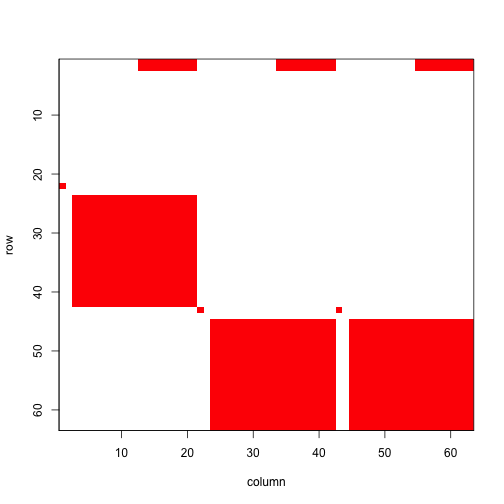Figure 8.4. Visualization of 1st A Matrix

#> []
#> NULL

The clear squares refer to zero elements, and the red elements refer to non-zero values corresponding to survival transitions and fecundity. The vast number of zeros may be surprising, but this matrix is a supermatrix organized by age first, with stage organizing within-age blocks. The first age is age 0, which cannot be adult, and age 1 corresponds to seedlings, leading to most non-zero elements in the adult portion. The adult block occurs from age 2, and this block can perpetuate indefinitely. The number of elements estimated is greater now than in the typical ahistorical MPM, because now we have added age as a major factor for analysis. This matrix is overwhelmingly composed of elements that must be 0, and so it is a rather sparse matrix ((726 + 36) / 3969 = 19.2% of elements).

We can see the order of ages and stages using the agestages element of the lefkoMat object we produced, as below. Note that our matrix is 63 rows by 63 columns, and this object gives us the exact order used.

#lathmat2age$agestages Now let’s estimate the element-wise arithmetic mean matrices. The first lefkoMat object created will include a single mean matrix, while the second will include six patch-level mean matrices followed by a grand mean matrix, yielding seven matrices (remember to look at the labels element of the output to see the exact order of matrices). lathmat2mean <- lmean(lathmat2age) lathmat2pmean <- lmean(lathmat2agep) #summary(lathmat2mean) #summary(lathmat2pmean) #### Step 5. MPM analysis Now let’s estimate the deterministic population growth rate $$\lambda$$, and the stochastic population growth rate, $$a = \text{log} \lambda _{S}$$, in our age x stage MPM. We’ll plot them for comparison, making sure to take the natural exponent of the stochastic growth rate to make it comparable to $$\lambda$$. We will show the patch means and the grand population mean. lambda2 <- lambda3(lathmat2age) lambda2m <- lambda3(lathmat2mean) lambda2mp <- lambda3(lathmat2pmean) set.seed(42) sl2 <- slambda3(lathmat2age) #Stochastic growth rate sl2$expa <- exp(sl2$a) par(mfrow = c(1,2)) plot(lambda ~ year2, data = lambda2, ylim = c(0.90, 0.98),xlab = "Year", ylab = expression(lambda), type = "l", lty= 1, lwd = 2, bty = "n") abline(a = lambda2m$lambda, b = 0, lty = 2, lwd= 2, col = "orangered")
abline(a = sl2$expa, b = 0, lty = 2, lwd= 3, col = "darkred") legend("bottomleft", c("det annual", "det mean", "stochastic"), lty = c(1, 2, 2), col = c("black", "orangered", "darkred"), lwd = c(2, 2, 3), bty = "n") plot(lambda ~ patch, data = lambda2mp[1:6,], ylim = c(0.90, 0.98), xlab = "Patch", ylab = expression(lambda), type = "l", lty= 1, lwd = 2, bty = "n") abline(a = lambda2m$lambda, b = 0, lty = 2, lwd= 2, col = "orangered")
abline(a = sl2$expa, b = 0, lty = 2, lwd= 2, col = "darkred") legend("bottomleft", c("patch det mean", "pop det mean", "pop sto"), lty = c(1, 2, 2), col = c("black", "orangered", "darkred"), lwd = 2, bty = "n")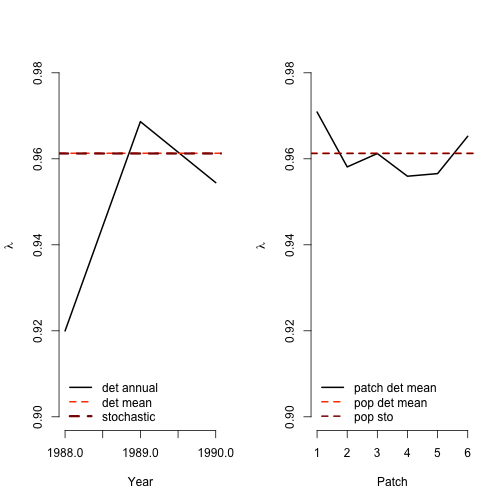Figure 8.5. Deterministic vs. stochastic lambda Deterministic and stochastic analyses suggest that the population and all patches are declining, with all years and patches associated with $$\lambda < 1$$ and $$a = \text{log} \lambda _{S} < 0$$. Qualitatively, they agree with the $$\lambda$$ and $$a = \text{log} \lambda _{S}$$ estimates from our other Lathyrus vignettes. Now let’s look at the stable stage distribution, using the population matrices rather than the patch matrices. The output for the ahistorical MPM is a data frame with matrix of origin, stage name, and stable stage proportion in each row. Because the default output for the stablestage3() function will give us the stable distribution of age-stages, we will collapse the output across age to produce a true stable stage distribution. ehrlen2ss <- stablestage3(lathmat2mean) ehrlen2ss_s <- stablestage3(lathmat2age, stochastic = TRUE, seed = 42) ss_props <- cbind.data.frame(ehrlen2ss$ss_prop, ehrlen2ss_s$ss_prop) names(ss_props) <- c("det", "sto") rownames(ss_props) <- paste(ehrlen2ss$age, ehrlen2ss$stage) det_dist <- apply(as.matrix(c(1:21)), 1, function(X) { ss_sum <- ss_props$det[X] + ss_props$det[21 + X] + ss_props$det[42 + X]
return(ss_sum)
})
sto_dist <- apply(as.matrix(c(1:21)), 1, function(X) {
ss_sum <- ss_props$sto[X] + ss_props$sto[21 + X] + ss_props$sto[42 + X] return(ss_sum) }) barplot(t(cbind.data.frame(det_dist, sto_dist)), beside = T, ylab = "Proportion", xaxt = "n", ylim = c(0, 0.2), col = c("black", "red"), bty = "n") text(cex=1, x=seq(from = 0.5, to = 3 * length(lathframeln$stage), by = 3),
y=-0.055, lathframeln$stage, xpd=TRUE, srt=45) legend("topright", c("deterministic", "stochastic"), col = c("black", "red"), pch = 15, bty = "n")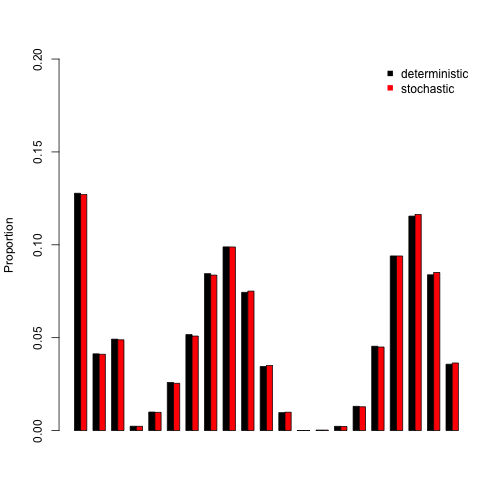Figure 8.6. Stable and long-run stage distribution The stable stage distribution shows high levels of dormant seeds and mid- to large-sized adults, followed by dormant adults. Small-sized adults comprise the smallest part of the stable age-stage structure. Deterministic and stochastic analyses show more or less the same results. Now let’s take look at the reproductive values. We’ll go straight to the plots, as with the stable stage distribution. ehrlen2rv <- repvalue3(lathmat2mean) ehrlen2rv_s <- repvalue3(lathmat2age, stochastic = TRUE, seed = 42) barplot(t(cbind.data.frame(ehrlen2rv$rep_value, ehrlen2rv_s$rep_value)), beside = T, ylab = "Relative rep value", xlab = "Age-Stage", ylim = c(0, 1.5), col = c("black", "red"), bty = "n") text(cex=0.5, y = -0.06, x = seq(from = 0, to = 2.98*length(rownames(ss_props)), by = 3), rownames(ss_props), xpd=TRUE, srt=45) legend("topleft", c("deterministic", "stochastic"), col = c("black", "red"), pch = 15, bty = "n")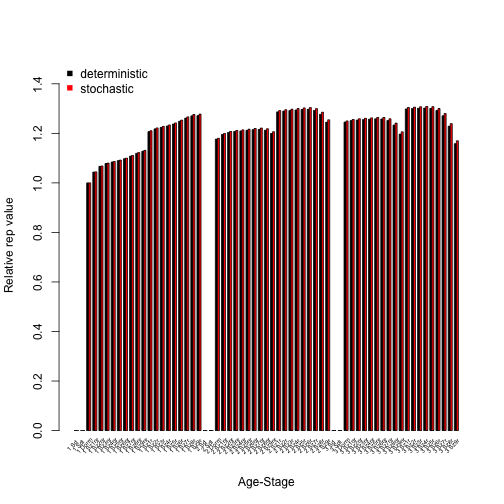Figure 8.7. Age-stage reproductive values We see reproductive values above 0 starting with dormant adults, and generally increasing into larger sizes with some interesting patterns in ages 2 and 3. These patterns hold in both deterministic and stochastic analyses. We will next assess to which matrix elements $$\lambda$$ is most sensitive and elastic to changes in. As before, we will look at both deterministic and stochastic sensitivities. lathmat2msens <- sensitivity3(lathmat2mean) set.seed(42) lathmat2msens_s <- sensitivity3(lathmat2age, stochastic = TRUE) writeLines(paste0("The highest deterministic sensitivity value: ", max(lathmat2msens$ah_sensmats[][which(lathmat2mean$A[] > 0)]))) #> The highest deterministic sensitivity value: 0.14396564929191 writeLines(paste0("This value is associated with element: ", which(lathmat2msens$ah_sensmats[] ==
max(lathmat2msens$ah_sensmats[][which(lathmat2mean$A[] > 0)]))))
#> This value is associated with element: 3838
writeLines(paste0("The highest stochastic sensitivity value: ",
max(lathmat2msens_s$ah_sensmats[][which(lathmat2mean$A[] > 0)])))
#> The highest stochastic sensitivity value: 0.151083270853861
writeLines(paste0("This value is associated with element: ",
which(lathmat2msens_s$ah_sensmats[] == max(lathmat2msens_s$ah_sensmats[][which(lathmat2mean$A[] > 0)])))) #> This value is associated with element: 3838 The highest deterministic sensitivity value in both analyses appears to be associated with the transition from non-flowering six-sprouted adults in age 3 or higher to flowering four-sprouted adult (element 3208, in column 51, row 58). The highest stochastic sensitivity value is assocaited with the transition from flowering seven-sprouted adult in age 3 or higher to flowering five-sprouted adult (element 3838, in column 61, row 59). Inspecting the sensitivity matrix (type lathmat2msens$ah_sensmats[] to view the full deterministic sensitivity matrix, or lathmat2msens_s$ah_sensmats[] to view the full stochastic sensitivity matrix) also shows that transitions near these elements are associated with rather high sensitivities. Let’s now assess the elasticity of $$\lambda$$ or $$\text{log} \lambda$$ to matrix elements. lathmat2melas <- elasticity3(lathmat2mean) set.seed(42) lathmat2melas_s <- elasticity3(lathmat2age, stochastic = TRUE) writeLines(paste0("The highest deterministic elasticity value: ", max(lathmat2melas$ah_elasmats[][which(lathmat2mean$A[] > 0)]))) #> The highest deterministic elasticity value: 0.0332105403473359 writeLines(paste0("The largest determnistic elasticity is associated with element: ", which(lathmat2melas$ah_elasmats[] == max(lathmat2melas$ah_elasmats[])))) #> The largest determnistic elasticity is associated with element: 3841 writeLines(paste0("The highest stochastic elasticity value: ", max(lathmat2melas_s$ah_elasmats[][which(lathmat2mean$A[] > 0)]))) #> The highest stochastic elasticity value: 0.033524701358656 writeLines(paste0("The largest stochastic elasticity is associated with element: ", which(lathmat2melas_s$ah_elasmats[] == max(lathmat2melas_s$ah_elasmats[])))) #> The largest stochastic elasticity is associated with element: 3841 Both deterministic and stochastic analyses show that population growth rate is most elastic to element 2820, which is in column 45 and row 48. Checking our stageframe shows that this element is associated with transition from dormant adult age 3 or higher to non-flowering three-sprouted adult. Elasticity values can be treated as additive and sum to 1.0 within single matrices. This allows us to make interesting comparisons, such as to compare the elasticity of population growth rate to changes in transitions associated with specific stages. Let’s conduct such an analysis, and plot the results. elas_put_together <- cbind.data.frame(colSums(lathmat2melas$ah_elasmats[]),
colSums(lathmat2melas_s$ah_elasmats[])) names(elas_put_together) <- c("det", "sto") rownames(elas_put_together) <- apply(as.matrix(c(1:dim(lathmat2melas$agestages))),
1, function(X) {
paste(lathmat2melas$agestages$stage[X], lathmat2melas$agestages$age[X])
})

barplot(t(elas_put_together), beside=T, names.arg = rep(NA,
length(rownames(elas_put_together))), ylab = "Elasticity", xlab = "Stage",
col = c("black", "orangered"), bty = "n")
text(cex=0.5, y = -0.007, x = seq(from = 0, to = 2.98*length(rownames(elas_put_together)),
by = 3), rownames(elas_put_together), xpd=TRUE, srt=45)
legend("topleft", c("deterministic", "stochastic"), col = c("black", "orangered"),
pch = 15, bty = "n")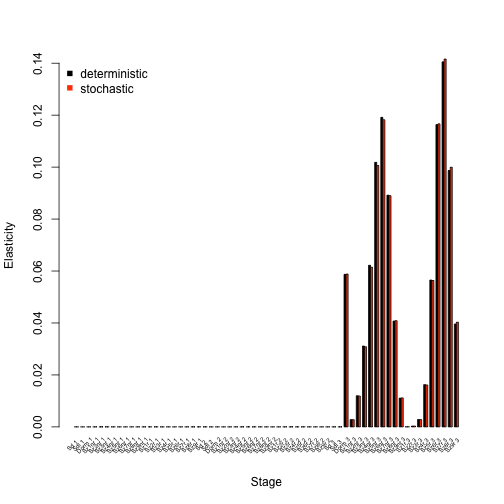Figure 8.8. Elasticities by age-stage

We see in the analysis above that in both the deterministic and stochastic cases, the population growth rate is most elastic to changes associated with flowering and non-flowering mid-sized adults, and with vegetatively dormant adults, in the highest ages. Juvenile stages and dormant seeds have little influence.

Our estimated elasticities can also be summarized by transition type. Let’s take a look at a plot, using the summary.lefkoElas() function to help us assess these transition types.

lathmat2m_sums <- summary(lathmat2melas)
lathmat2m_s_sums <- summary(lathmat2melas_s)

elas_sums_together <- cbind.data.frame(lathmat2m_sums$ahist[,2], lathmat2m_s_sums$ahist[,2])
names(elas_sums_together) <- c("det", "sto")
rownames(elas_sums_together) <- lathmat2m_sums$ahist$category

barplot(t(elas_sums_together), beside=T, ylab = "Elasticity", xlab = "Transition",
col = c("black", "orangered"), bty = "n")
legend("topright", c("deterministic", "stochastic"), col = c("black", "orangered"),
pch = 15, bty = "n")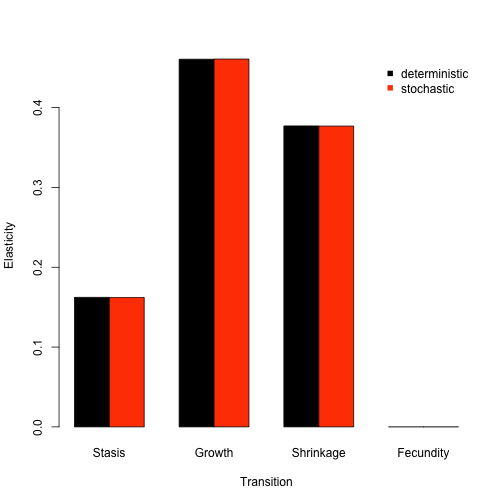Figure 8.9. Elasticities by transition type

We see in the plot above that deterministic and stochastic analyses agree strongly about the importance and influence of transition types to population growth rate. Specifically, the population growth rate is most strongly elastic in response to changes in growth transitions, and almost completely inelastic to changes in fecundity. Shrinkage is also fairly influential.

In addition to sensitivity and elasticity analyses, we can use package lefko3 to assess the actual impacts of demographic shifts or differences on the population growth rate. Two tools for this purpose are the life table response experiment (LTRE), and its stochastic version the stochastic life table response experiment (sLTRE). Both are available via the ltre3() function. Below, we perform a standard deterministic LTRE to assess the impact of space on the population growth rate. This is done via a comparison of patch-level demography to the grand mean matrix, which is the default comparison if no reference matrices are provided. Remove the hashtag from the second line to see the full structure of the resulting object. Particularly, you will notice that the first element, ltre_det, is a list of six matrices. These matrices show the actual impact of the difference in elements between the respective patch-level matrix and the reference matrix (here, the grand population mean matrix) on the population growth rate $$\lambda$$. After this, we see similar structure to the input lefkoMat object, including the stageframe and order of matrices.

lathmat2m_ltre <- ltre3(lathmat2pmean)
#> Warning: Matrices input as mats will also be used as reference matrices.
#> Using all refmats matrices as reference matrices.
#lathmat2m_ltre

The sLTRE produces output that is a bit different. Here, we see two lists of matrices prior to the MPM metadata. The first, ltre_mean, is a list of matrices showing the impact of differences in mean elements between the patch-level temporal mean matrices and the reference temporal mean matrix. The second, ltre_sd, is a list of matrices showing the impact of differences in the temporal standard deviation of each element between the patch-level and reference matrix sets. In other words, while a standard LTRE shows the impact of changes in matrix elements on $$\lambda$$, the sLTRE shows the impacts of changes in the mean and the variability of matrix elements on $$\text{log} \lambda$$.

lathmat2m_sltre <- ltre3(lathmat2agep, stochastic = TRUE, rseed = 42)
#> Warning: Matrices input as mats will also be used as reference matrices.
#> Using all refmats matrices as reference matrices.
#lathmat2m_sltre

The output objects are large and can take a great deal of effort to look over and understand. Therefore, we will show three approaches to assessing these objects, using an approach similar to that used to assess elasticities. These methods can be used to assess patterns in all six patches, but for brevity we will focus only on the first matrix here. First, we will identify the elements most strongly impacting the population growth rate in each case.

writeLines(paste0("Highest (i.e most positive) deterministic LTRE contribution: ",
max(lathmat2m_ltre$ltre_det[]))) #> Highest (i.e most positive) deterministic LTRE contribution: 0.00184635504067327 writeLines(paste0("Highest deterministic LTRE contribution is associated with element: ", which(lathmat2m_ltre$ltre_det[] == max(lathmat2m_ltre$ltre_det[])))) #> Highest deterministic LTRE contribution is associated with element: 3841 writeLines(paste0("Lowest (i.e. most negative) deterministic LTRE contribution: ", min(lathmat2m_ltre$ltre_det[])))
#> Lowest (i.e. most negative) deterministic LTRE contribution: -0.00235987126640427
writeLines(paste0("Lowest deterministic LTRE contribution is associated with element: ",
which(lathmat2m_ltre$ltre_det[] == min(lathmat2m_ltre$ltre_det[]))))
#> Lowest deterministic LTRE contribution is associated with element: 3825

writeLines(paste0("Highest stochastic mean LTRE contribution: ",
max(lathmat2m_sltre$ltre_mean[]))) #> Highest stochastic mean LTRE contribution: 0.00176132067911133 writeLines(paste0("Highest stochastic mean LTRE contribution is associated with element: ", which(lathmat2m_sltre$ltre_mean[] == max(lathmat2m_sltre$ltre_mean[])))) #> Highest stochastic mean LTRE contribution is associated with element: 3841 writeLines(paste0("Lowest stochastic mean LTRE contribution: ", min(lathmat2m_sltre$ltre_mean[])))
#> Lowest stochastic mean LTRE contribution: -0.00267096555627256
writeLines(paste0("Lowest stochastic mean LTRE contribution is associated with element: ",
which(lathmat2m_sltre$ltre_mean[] == min(lathmat2m_sltre$ltre_mean[]))))
#> Lowest stochastic mean LTRE contribution is associated with element: 3825

writeLines(paste0("Highest stochastic SD LTRE contribution: ",
max(lathmat2m_sltre$ltre_sd[]))) #> Highest stochastic SD LTRE contribution: 3.37901045579132e-05 writeLines(paste0("Highest stochastic SD LTRE contribution is associated with element: ", which(lathmat2m_sltre$ltre_sd[] == max(lathmat2m_sltre$ltre_sd[])))) #> Highest stochastic SD LTRE contribution is associated with element: 3138 writeLines(paste0("Lowest stochastic SD LTRE contribution: ", min(lathmat2m_sltre$ltre_sd[])))
#> Lowest stochastic SD LTRE contribution: -2.95205258298888e-05
writeLines(paste0("Lowest stochastic SD LTRE contribution is associated with element: ",
which(lathmat2m_sltre$ltre_sd[] == min(lathmat2m_sltre$ltre_sd[]))))
#> Lowest stochastic SD LTRE contribution is associated with element: 3841

writeLines(paste0("Total positive deterministic LTRE contributions: ",
sum(lathmat2m_ltre$ltre_det[][which(lathmat2m_ltre$ltre_det[] > 0)])))
#> Total positive deterministic LTRE contributions: 0.0341116763064364
writeLines(paste0("Total negative deterministic LTRE contributions: ",
sum(lathmat2m_ltre$ltre_det[][which(lathmat2m_ltre$ltre_det[] < 0)])))
#> Total negative deterministic LTRE contributions: -0.0255192566804928
writeLines(paste0("\nTotal positive stochastic mean LTRE contributions: ",
sum(lathmat2m_sltre$ltre_mean[][which(lathmat2m_sltre$ltre_mean[] > 0)])))
#>
#> Total positive stochastic mean LTRE contributions: 0.0342840015465079
writeLines(paste0("Total negative stochastic mean LTRE contributions: ",
sum(lathmat2m_sltre$ltre_mean[][which(lathmat2m_sltre$ltre_mean[] < 0)])))
#> Total negative stochastic mean LTRE contributions: -0.0290170479685542
writeLines(paste0("\nTotal positive stochastic SD LTRE contributions: ",
sum(lathmat2m_sltre$ltre_sd[][which(lathmat2m_sltre$ltre_sd[] > 0)])))
#>
#> Total positive stochastic SD LTRE contributions: 0.000288501420787641
writeLines(paste0("Total negative stochastic SD LTRE contributions: ",
sum(lathmat2m_sltre$ltre_sd[][which(lathmat2m_sltre$ltre_sd[] < 0)])))
#> Total negative stochastic SD LTRE contributions: -0.000580097782208445

All LTRE outputs show very small contributions from individual elements. However, element 3841 appears to be the most positive in both the deterministic and stochastic mean cases. This is the stasis transition for seven-sprouted flowering adults. Variability in elements appears to have much smaller impact on $$log \lambda$$ than shifts in the means do.

Second, we will identify which age-stages exert the strongest impact on the population growth rate.

ltre_pos <- lathmat2m_ltre$ltre_det[] ltre_neg <- lathmat2m_ltre$ltre_det[]
ltre_pos[which(ltre_pos < 0)] <- 0
ltre_neg[which(ltre_neg > 0)] <- 0

sltre_meanpos <- lathmat2m_sltre$ltre_mean[] sltre_meanneg <- lathmat2m_sltre$ltre_mean[]
sltre_meanpos[which(sltre_meanpos < 0)] <- 0
sltre_meanneg[which(sltre_meanneg > 0)] <- 0

sltre_sdpos <- lathmat2m_sltre$ltre_sd[] sltre_sdneg <- lathmat2m_sltre$ltre_sd[]
sltre_sdpos[which(sltre_sdpos < 0)] <- 0
sltre_sdneg[which(sltre_sdneg > 0)] <- 0

ltresums_pos <- cbind(colSums(ltre_pos), colSums(sltre_meanpos), colSums(sltre_sdpos))
ltresums_neg <- cbind(colSums(ltre_neg), colSums(sltre_meanneg), colSums(sltre_sdneg))

ltre_as_names <- apply(as.matrix(c(1:length(lathmat2agep$agestages$stage))), 1,
function(X) {
paste(lathmat2agep$agestages$stage[X], lathmat2agep$agestages$age[X])
})
barplot(t(ltresums_pos), beside = T, col = c("black", "grey", "red"),
ylim = c(-0.006, 0.006))
barplot(t(ltresums_neg), beside = T, col = c("black", "grey", "red"), add = TRUE)
text(cex=0.5, y = -0.0065, x = seq(from = 0, to = 3.98*length(ltre_as_names),
by = 4), ltre_as_names, xpd=TRUE, srt=45)
legend("topleft", c("deterministic", "stochastic mean", "stochastic SD"),
col = c("black", "grey", "red"), pch = 15, bty = "n")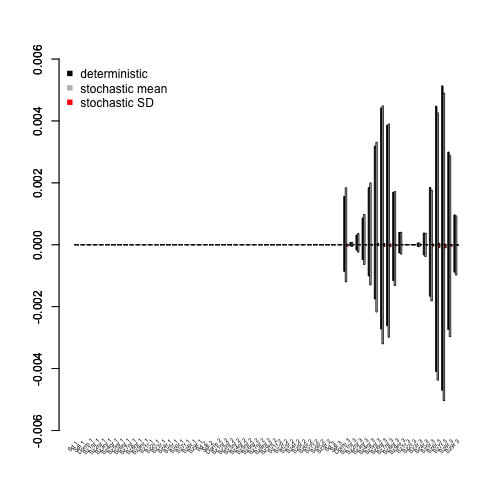Figure 8.10. LTRE contributions by age-stage

The output above shows that mid-sized non-flowering and flowering adults and seedlings exert strong influences on both $$\lambda$$ and $$\text{log} \lambda$$.

Finally, we will assess what transition types exert the greatest impact on population growth rate.

lathmat2m_ltre_summary <- summary(lathmat2m_ltre)
lathmat2m_sltre_summary <- summary(lathmat2m_sltre)

ltresums_tpos <- cbind(lathmat2m_ltre_summary$ahist_det$matrix1_pos,
lathmat2m_sltre_summary$ahist_mean$matrix1_pos,
lathmat2m_sltre_summary$ahist_sd$matrix1_pos)
ltresums_tneg <- cbind(lathmat2m_ltre_summary$ahist_det$matrix1_neg,
lathmat2m_sltre_summary$ahist_mean$matrix1_neg,
lathmat2m_sltre_summary$ahist_sd$matrix1_neg)

barplot(t(ltresums_tpos), beside = T, col = c("black", "grey", "red"),
ylim = c(-0.03, 0.03))
barplot(t(ltresums_tneg), beside = T, col = c("black", "grey", "red"),
abline(0, 0, lty = 3)
text(cex=0.85, y = -0.040, x = seq(from = 2,
to = 3.98*length(lathmat2m_ltre_summary$ahist_det$category), by = 4),
lathmat2m_ltre_summary$ahist_det$category, xpd=TRUE, srt=45)
legend("topleft", c("deterministic", "stochastic mean", "stochastic SD"),
col = c("black", "grey", "red"), pch = 15, bty = "n")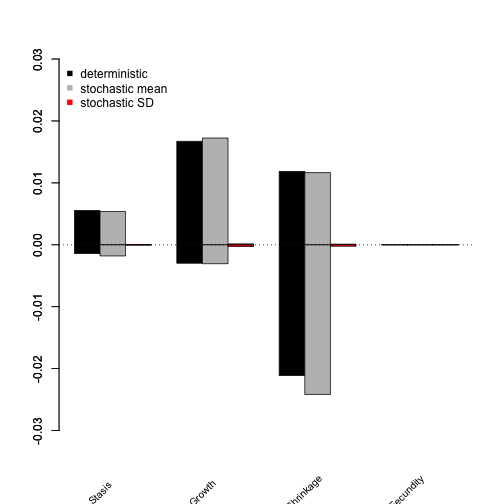Figure 8.11. LTRE contributions by transition type

The overall greatest impact on the population growth rate appears to come from growth and shrinkage transitions, with shrinkage transitions having strongly negative influences in general.

LTREs and sLTREs are powerful tools, and more complex versions of both analyses exist. Please consult Caswell (2001) and Davison et al. (2013) for more information. Package lefko3 also includes functions complex analyses, including two general projection functions that can be used for analyses such as quasi-extinction analysis as well as for density dependent analysis. Users wishing to conduct these analyses should see our free e-manual called lefko3: a gentle introduction and other vignettes, including long-format and video vignettes, on the projects page of the Shefferson lab website.

## Acknowledgements

We are grateful to two anonymous reviewers whose scrutiny improved the quality of this vignette. The project resulting in this package and this tutorial was funded by Grant-In-Aid 19H03298 from the Japan Society for the Promotion of Science.

## Literature cited

Bartoń, Kamil A. 2014. MuMIn: Multi-Model Inference.” https://CRAN.R-project.org/package=MuMIn.
Bates, Douglas, Martin Maechler, Ben Bolker, and Steve Walker. 2015. “Fitting Linear Mixed-Effects Models Using Lme4.” Journal of Statistical Software 67 (1): 1–48. https://doi.org/10.18637/jss.v067.i01.
Brooks, Mollie E., Kasper Kristensen, Koen J. van Benthem, Arni Magnusson, Casper W. Berg, Anders Nielsen, Hans J. Skaug, Martin Machler, and Benjamin M. Bolker. 2017. glmmTMB Balances Speed and Flexibility Among Packages for Zero-Inflated Generalized Linear Mixed Modeling.” The R Journal 9 (2): 378–400. https://doi.org/10.32614/RJ-2017-066.
Caswell, Hal. 2001. Matrix Population Models: Construction, Analysis, and Interpretation. Second edition. Sunderland, Massachusetts, USA: Sinauer Associates, Inc.
Davison, Raziel, Florence Nicole, Hans Jacquemyn, and Shripad Tuljapurkar. 2013. “Contributions of Covariance: Decomposing the Components of Stochastic Population Growth in Cypripedium Calceolus.” The American Naturalist 181 (3): 410–20. https://doi.org/10.1086/669155.
Doak, Daniel F., Ellen Waddle, Ryan E. Langendorf, Allison M. Louthan, Nathalie Isabelle Chardon, Reilly R. Dibner, Douglas A. Keinath, et al. 2021. “A Critical Comparison of Integral Projection and Matrix Projection Models for Demographic Analysis.” Ecological Monographs 91 (2): e01447. https://doi.org/10.1002/ecm.1447.
Ehrlén, Johan. 1995. “Demography of the Perennial Herb Lathyrus Vernus. I. Herbivory and Individual Performance.” Journal of Ecology 83 (2): 287–95. https://doi.org/10.2307/2261567.
———. 2000. “The Dynamics of Plant Populations: Does the History of Individuals Matter?” Ecology 81 (6): 1675–84. https://doi.org/10.1890/0012-9658(2000)081[1675:TDOPPD]2.0.CO;2.
———. 2002. “Assessing the Lifetime Consequences of Plant-Animal Interactions for the Perennial Herb Lathyrus Vernus (Fabaceae).” Perspectives in Plant Ecology, Evolution and Systematics 5 (3): 145–63. https://doi.org/10.1078/1433-8319-00031.
Ehrlén, Johan, and Ove Eriksson. 1996. “Seedling Recruitment in the Perennial Herb Lathyrus Vernus.” Flora 191 (4): 377–83. https://doi.org/10.1016/S0367-2530(17)30744-2.
Ehrlén, Johan, and Kari Lehtila. 2002. “How Perennal Are Perennial Plants?” Oikos 98: 308–22. https://doi.org/10.1034/j.1600-0706.2002.980212.x.
Ehrlén, Johan, and Zuzana Munzbergova. 2009. “Timing of Flowering: Opposed Selection on Different Fitness Components and Trait Covariation.” The American Naturalist 173 (6): 819–30. https://doi.org/10.1086/598492.
Ehrlén, Johan, and Jan Van Groenendael. 2001. “Storage and the Delayed Costs of Reproduction in the Understorey Perennial Lathyrus Vernus.” Journal of Ecology 89 (2): 237–46. https://doi.org/10.1046/j.1365-2745.2001.00546.x.